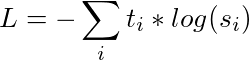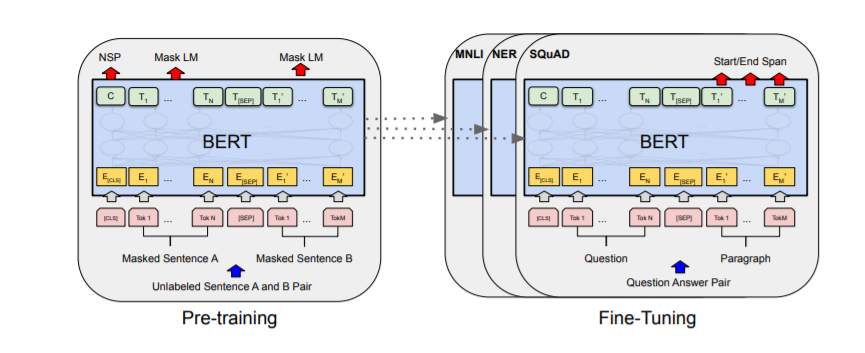Drishti Sharma — Published On November 3, 2022 and Last Modified On November 11th, 2022

Source: Canva

## Introduction

In 2018, GoogleAI researchers released the BERT model. It was a fantastic work that brought a revolution in the NLP domain. However, the BERT model did have some drawbacks i.e. it was bulky and hence a little slow. To navigate these issues, researchers from Hugging Face proposed DistilBERT, which employed knowledge distillation for model compression.

In this article, we will look at this work in more detail.

Now, let’s begin!

## DistilBERT Highlights

• DistilBERT model is a distilled form of the BERT model. The size of a BERT model was reduced by 40% via knowledge distillation during the pre-training phase while retaining 97% of its language understanding abilities and being 60% faster.

• A triple loss is introduced by combining language modeling, distillation, and cosine-distance losses to take advantage of the inductive biases learned by larger models during pre-training.

• DistilBERT is a compact, faster, and lighter model that is cheaper to pre-train and can easily be used for on-device applications.

## Why Do We Need a DistilBERT-like Model?

In recent years the transfer learning approach with large pre-trained models has become mainstream. Although these large-scale models lead to a significant gain in performance, these models often have millions of parameters. Recent studies on pre-trained models suggest that training even larger models still results in improved results on downstream tasks. And the following reasons make this trend toward bigger models a matter of concern:

1. The environmental cost of scaling a large-scale model is concerning considering these models’ computational requirements.

2. Operating these large and bulky models in on-the-edge or limited constraint settings (resources like limited computing tools, budget, etc.) is challenging for training and inferencing, which could hamper wide adoption.

3. To develop more privacy-respecting systems, machine learning systems must operate on the edge rather than accessing a cloud API and transferring potentially private data to servers. Running models on smartphones also requires lightweight, energy-efficient, and responsive models!

To navigate the aforementioned challenges, model compression techniques can be leveraged. For that, there are many techniques like quantization (approximating the weights of a network with a smaller precision), knowledge distillation (teacher-student learning), and weights pruning (removing some connections in the network). However, the key focus of DistilBERT is on knowledge distillation.

## What is Knowledge Distillation & How Does It Benefit the Student Model?

Knowledge Distillation (also known as teacher-student learning) is a model compression technique in which a smaller model (the student model) is trained to reproduce the capabilities of a larger model (the teacher model) or an ensemble of models.

In supervised learning, a classification model is usually trained to predict a class by maximizing the estimated probability of gold labels. A standard training objective constitutes decreasing the cross-entropy between the one-hot empirical distribution of training labels and the model’s predicted distribution. A well-trained model predicts an output distribution with a high probability on the correct class and almost zero (non-zero) probabilities on other classes. However, some of these non-zero probabilities are greater than others, reflected in the model’s generalization capabilities or performance.

For instance, a lab coat might be mistaken for a shirt but should usually not be mistaken for an apple. Another way to think about distillation is that it keeps the model from being overly confident in its predictions (similar to label smoothing).

In knowledge distillation, the student model is trained to mimic the output distribution of the parent model (i.e. its knowledge). Instead of training with a cross-entropy over the hard targets, the knowledge is passed from the teacher model to the student with a cross-entropy over the soft targets. The training loss thus becomes:where ti is the probability estimated by the teacher and si is the probability estimated by the student.

This loss is a stronger/richer training signal since a single example enforces a lot more constraint than a single hard target.

To clearly show the mass of the distribution over the classes, a softmax temperature is defined as:where T is the temperature parameter that regulates the smoothness of the output distribution and zi
represents the model score for class i.

Case 1: When T → 0, the distribution takes on the form of Kronecker (and is analogous to the one-hot target vector)

Case 2: When T →+∞, it becomes a uniform distribution.

During training, the teacher and the student models are subjected to the same temperature setting. At inference, T is initialized to 1 and recovers the standard Softmax.

The final training objective is a linear combination of the supervised training loss (i.e. masked language modeling loss Lmlm) and the distillation loss Lce. Additionally, adding a cosine embedding loss (Lcos), which tends to align the directions of the student and teacher hidden states vectors, was advantageous.

## DistilBERT Method Overview

DistilBERT (the student) has the same general architecture as BERT (the teacher) except that the token-type embeddings and pooler are removed along with a 2x reduction in the number of layers.Considering the majority of the operations used in the Transformer architecture (linear layer and layer normalization) are highly optimized, investigations suggested that variations on the last dimension of the tensor (hidden size dimension) have little effect on computation efficiency than variations on other factors e.g., the number of layers. Thus the prime focus was on reducing the number of layers.

Apart from the previously discussed architectural choices and optimization, a crucial step in the training process is finding the optimal initialization for the converging sub-network. In this regard, by leveraging the common dimensionality between the teacher and the student networks, the student network is initialized from the teacher network by taking one layer out of two.

In DistilBERT, the best practices of training the BERT model are incorporated. DistilBERT is distilled using huge batches with the help of gradient accumulation using dynamic masking and without the next sentence prediction (NSP) objective.

DistilBEERT was also trained on the same dataset as the original BERT model i.e., English Wikipedia and Toronto Book Corpus. Moreover, it was trained on 8 16GB V100 GPUs for about 90 hours, whereas the RoBERTa model took 1 day to train on 1024 32GB V100.

## Evaluation Results

1. Language Understanding and Generalization Capabilities: The language understanding and generalization capabilities of DistilBERT were assessed on GLUE benchmark (a collection of 9 datasets/tasks). On testing, it was found that the DistilBERT always compares favorably to or outperforms the ELMo baseline. Also, DistilBERT outperforms BERT by retaining 97% of the performance while using 40% fewer parameters.

2. Performance of DistilBERT on downstream tasks: DistilBERT is behind BERT by only 0.6% points in test accuracy on the IMDb benchmark while being 40% smaller. Moreover, DistilBERT is also behind BERT by some points on SQuaD.

Table 1: Performance of DistilBERT on downstream tasks

3. Speed-up/Size trade-off of DistilBERT: To investigate this, the number of parameters of each model and the inference time required to do a full pass on the STSB development set on a CPU were compared. DistilBERT has 40% fewer parameters and is around 60% faster than BERT.

4. On-device Computation: On comparing the average inference time of a DistilBERT-based mobile application for question-answering (on iPhone 7 plus) with a previously trained question-answering model-based on BERT-base, it was found that neglecting the tokenization step, DistilBERT is around 71% faster than BERT and model weighs only 207 MB.

## How to Use DistilBERT in Projects?

Huggingface’s Transformers library offers a variety of DistilBERT models in different versions and sizes. In this post, we will focus on loading the model and performing single-label classification for demonstration purposes.

We will first install and import all necessary packages and load the model  (“distilbert-base-uncased-finetuned-sst-2-english”) and its tokenizer from DistilBertForSequenceClassification and DistilBertTokenizer, respectively. And then, we will pass the input through the tokenizer to extract tokenized output which will be subsequently utilized for predicting the label (in our case, sentiment).

`!pip install -q transformers`
```import torch
from transformers import DistilBertTokenizer, DistilBertForSequenceClassification```
```tokenizer = DistilBertTokenizer.from_pretrained("distilbert-base-uncased-finetuned-sst-2-english")
model = DistilBertForSequenceClassification.from_pretrained("distilbert-base-uncased-finetuned-sst-2-english")```
`inputs = tokenizer("Wow! What a surprise!", return_tensors="pt")`
```with torch.inference_mode():
logits = model(**inputs).logits```
```predicted_class_id = logits.argmax().item()
model.config.id2label[predicted_class_id]```

>> Output: “POSITIVE”

## Conclusion

So in this article, we saw how BERT could be distilled to obtain a compact, lighter and faster model called BERT, which can find applications in an on-device setup.

1. In recent years, the transfer learning approaches in Natural Language Preprocessing with large pre-trained models have become mainstream. Model compression techniques could be leveraged to produce these massive/bulky models.
2. DistilBERT model is a distilled form of the BERT model. The size of a BERT model was reduced by 40% via knowledge distillation during the pre-training phase while retaining 97% of its language understanding abilities and being 60% faster.
3. Knowledge Distillation (also known as teacher-student learning) is a model compression technique in which a smaller model (the student model) is trained to reproduce the capabilities of a larger model (the teacher model) or an ensemble of models.
4. The student model is trained to generalize the same way as the teacher model by matching the output distribution (i.e. the teacher model’s knowledge).
5. A triple loss is introduced by combining language modeling, distillation, and cosine-distance losses to take advantage of the inductive biases learned by larger models during pre-training.
6. DistilBERT is a compact, faster, and lighter model that is cheaper to pre-train and can be used for on-device applications with competent performance results.